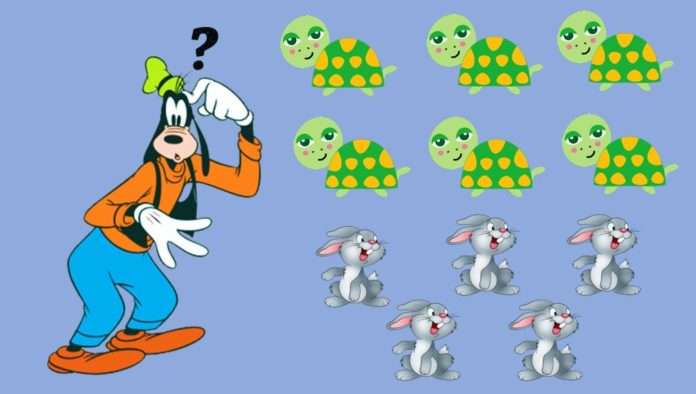# Ratio and Proportion Questions for Bank PO

0
2551## RATIO AND PROPORTION FOR BANK PO

The Notification for SBI PO is out . Since, the SBI PO Exam is very competitive, the Expected Cutoff for SBI PO remains a mystery. If we go through SBI PO Previous Papers, it is evident that Ratio and Proportion questions for Bank PO act as a game changer. Ratio and Proportion Questions for Bank PO Exams are asked under the Quantitative Aptitude Section.

There are 2-3 questions on this topic which have difficulty level from Easy to Moderate. Since, Banking Exams are very competitive and even minute difference can matter in the long run it is necessary to prepare Ratio and Proportion questions for Bank PO by attempting Questions in free SBI PO mock tests. You can find amazing tips and shortcut tricks below to tackle the questions related to Ratio and Proportion during PO Examination.

## RATIO

Ratio is defined as the number of times one quantity contains the other quantity. The mathematical symbol for the ratio is “:”. We should note that both the quantities must have same units or types. If there 20 mangoes and 30 apples in a basket, we can say that the ratio of mangoes to apples is 2:3 as both the quantities represent the fruits in the basket. But, we cannot say the ratio of Rs.40 to 50 apples is 4:5 as both are different items.

The term written on the left of “:” is known as antecedent and the term written on the right of “:” is known as consequent. Since, both the quantities have same units, the ratio does not have a unit.

### TERMS ASSOCIATED WITH RATIO

• Inverse or Reciprocal of Ratio: If the given ratio is 3:4 or $$\frac{3}{4}$$ , then the inverse or reciprocal is written as 4:3 or $$\frac{4}{3}$$
• Equality of Ratio: If antecedent and consequent of a ratio are equal. For e.g. 5:5
• Lesser Inequality of Ratio: If the antecedent is lesser than consequent. For e.g. 3:4
• Greater Inequality of Ratio: If the antecedent is greater than consequent. For e.g. 5:4

Example: Divide 729 into two parts such that the ratio between the two is 5:4

1st Part = $$\frac{5\times 729}{5 + 4}$$ = 405

2nd Part = $$\frac{4\times 729}{5 + 4}$$ = 324

Note that the ratio 405:324 remains same as 5:4 and have a common factor as 81

## PROPORTION

The ratios are said to be in proportion if two or more ratios are equal. Let the 1st ratio be 3:9 and 2nd ratio be 9:27. Since , 3 is one-third of 9 and 9 is one-third of 27. Both the ratios are equal, this is called equality of ratio or proportion. Proportion is written with the symbol “::” or “=” . Therefore , proportion can be written as 3 : 9 :: 9 : 27 or 3 : 9 = 9 : 27 or 3/9 = 9/27 . The terms at the end are known as extremes, and the terms in the middle are known as means.

### PROPERTIES OF PROPORTION

• ###### Product of Means is equal to the Product of Extremes:

Lets say, quantities 5, 7, 15 and 21 are in proportion such 5 : 7 = 15 : 21 then (5×21) is equal to (15×7) , i.e. 105

## EXAMPLES OF RATIO AND PROPORTION QUESTIONS FOR BANK PO EXAMS

Example 1: If the price of 6 balls is Rs.11, then find the price of 18 balls?

Solution: If the quantity of balls is increased by a factor , the price also gets increased by that much factor . Therefore , 6 balls : 18 balls = Rs. 11 : Rs. x $$\implies$$ $$\frac{6}{18}$$ = $$\frac{11}{x}$$ $$\implies$$ x = Rs.33

Example 2: If 20 men can do work in 30 days, then how many days would 10 men require?

Solution: This is an example of inverse ratio , as the number of men decreases , the days required to complete the work would increase . Therefore , $$\frac{1}{20}$$ : $$\frac{1}{10}$$ = $$30$$ : $$x$$ $$\implies$$ $$\frac{x}{20}$$ = $$\frac{1}{10}\times 30$$ $$\implies$$ $$x$$ = $$60$$ days . In above relation , one quantity is multiplied by a factor , the other quantity is divided by that factor .

Example 3: If a company reduces its workforce by the ratio 10:9 and increases the salary by the ratio 12:13, find the ratio of expenditure?

Solution: The ratio of the expenditures would be (10 x 12) : (9 x 13) = 120:117 = 40:39

Example 4: If a 60 ml of water contains 12% of chlorine, how much water must be added to create a 8% chlorine solution?

Solution: Amount of Chlorine in the mixture , $$\frac{12}{100}$$ = $$\frac{x}{60}$$ $$\implies$$ $$x$$ = $$\frac{12 \times 60}{100}$$ = 7.2 ml
Now , when we add only water , the amount of Chlorine in the solution remains same but the ratio will change . Therefore , $$\frac{8}{100}$$ = $$\frac{7.2}{z}$$ $$\implies$$ $$z$$ = $$\frac{7.2\times100}{8}$$ $$\implies$$ $$z$$ = $$90$$ . So , the volume of mixture has changed from 60 ml to 90 ml . The amount of water added , $$V$$ = $$( 90 – 60 )$$ ml = $$30$$ ml

We have Blogs on other topics as well to help you ace Bank PO Examination
Data Interpretation for SBI PO
Number System for SBI PO exam